Latest Banking jobs   »

# Quantitative Aptitude Quiz For SIDBI Grade A Prelims 2023- 25th January

Q1. Ratio of present age of A to that of B is 1 : 3 and one year ago, C is twice as old as A. If B is 21 years older than C, then find present age of B & C together is what percent of present age of A?
(a) 545%
(b) 480%
(c) 500%
(d) 525%
(e) 495%

Q2. How many 7 letters word can be formed from the word “GATEWAY” such that the vowels occupy the extreme ends?
(a) 72 ways
(b) 720 ways
(c) 180 ways
(d) 360 ways
(e) 144 ways

Q3. Deepak and Dharam entered into a partnership and invested Rs.40000 and Rs.55000 respectively. After 10 months and 8 months Deepak and Dharam withdraws his amount from the business and after 6 months Veer invested Rs.60000. If at the end of the year difference in the profit share of Deepak and Dharam is Rs.5225, then find profit share of Veer at the end of the year.
(a) Rs.49560
(b) Rs.47025
(c) Rs.48235
(d) Rs.48970
(e) Rs.49255

Q4. A shopkeeper sells chairs and tables and cost price of a table is Rs.3400 more than cost price of a chair. Shopkeeper marks table and chair 50% and 80% above the cost price respectively and he allowed 25% discount on a chair and 32% discount on a table. If profit earned by shopkeeper on selling a chair is Rs.130 more than profit earned by him on selling a table, then find difference between selling price of a table and a chair.
(a) Rs.3560
(b) Rs.3270
(c) Rs.3340
(d) Rs.3480
(e) Rs.3510

Q5. (T+ 4) type ‘A’ types of pipes can fill a tank in 2T hours while (T +12) type ‘B’ types of pipes can fill the same tank in (T + 8) hours. If ratio of efficiency of type ‘A’ to type ‘B’ pipe is 5 : 4, then find in how many hours 12 type ‘A’ and 15 type ‘B’ pipes together can fill the same tank?
(a) 18 hours
(b) 20 hours
(c) 24 hours
(d) 16 hours
(e) 12 hours

Q6. Ankit bought an article at Rs.1200. He marks up the cost of article and sells it in such a way that if he will give 46 ⅔%discount, then loss occur to him is equal to profit earn by him when he will give 20% discount. Find the Mark price of article?
(a) Rs. 1600
(b) Rs. 1400
(c) Rs. 1200
(d) Rs. 1000
(e) Rs. 1800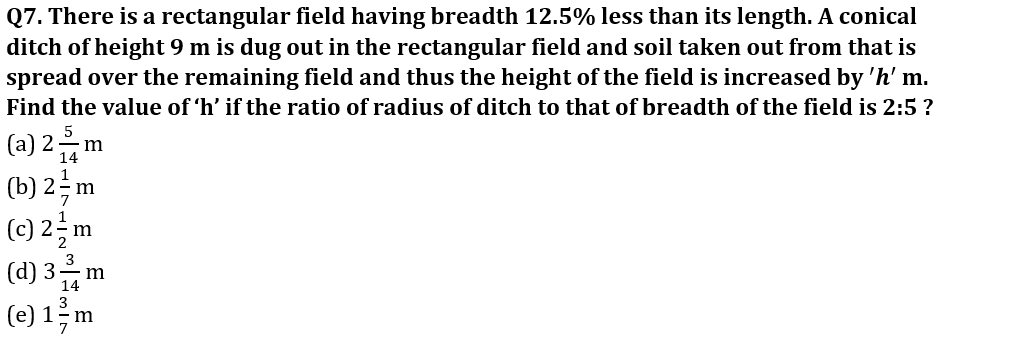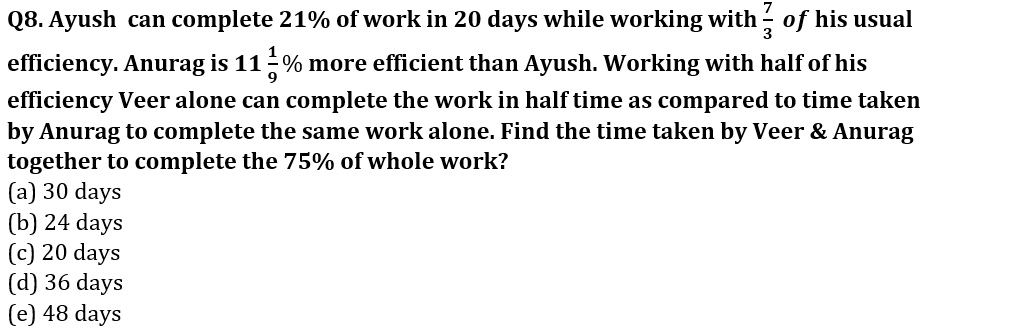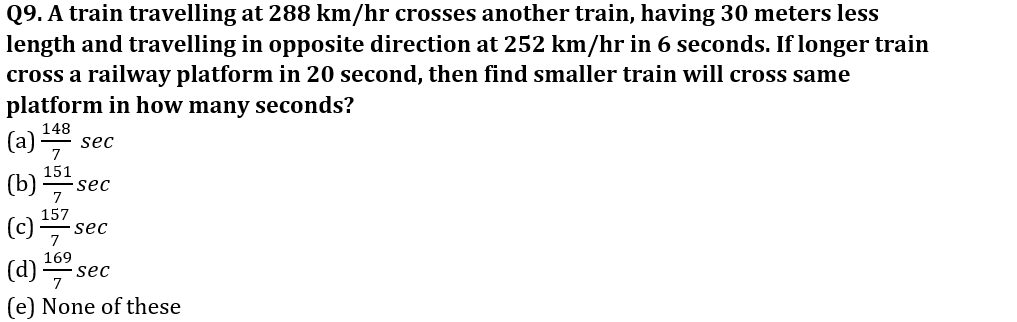Q10. Sameer invested his saving of Rs. 1 lakh in two schemes for two years, first scheme offers him rate of interest of 10% on CI and other offers him rate of interest of 15% on SI. If interest obtained by him in former scheme is Rs. 600 more than that of later, find the amount invested by him in later scheme.
(a) Rs. 40000
(b) Rs. 24000
(c) Rs. 48000
(d) Rs. 56000
(e) None of these

Q11. 2n years ago, the age of Raju was four times that of his son and n years ago, the age of Raju was thrice that of his son. If n years later, the sum of the ages of Raju and his son will be 80 years, then the difference in the ages of Raju and his son is
(a) 20 years
(b) 40 years
(c) 24 years
(d) 30 years
(e) 35 years
Q12. Vessel X contains (Q + 36) liter mixture of milk & water in the ratio of 7: 2, while vessel Y contains (2Q + 42) liter mixture of milk & water in the ratio of 2 : 3. If 40% & 46% liter mixture from vessel X and Y taken out respectively, then mixture remaining in vessel Y is 150% of mixture remaining in vessel X. Find the total initial quantity of milk in mixture of vessel X & vessel Y together? (in liters)
(a)130
(b)180
(c)90
(d)150
(e)210

Q13. In a bucket there are different colored balls of black, blue and white colors. The probability of selecting one black ball out of the total ball is 2/5 and the probability of selecting one blue ball out of the total ball is 3/7.The number of white balls in the bag is 18. Find total number of balls in the bucket?
(a)135
(b)80
(c)120
(d)90
(e)105

Q14. A sugar solution has 60% water. If 2.5 kg of water evaporates from 10 kg of the solution and 2.5 kg of initial sugar solution is added again to remaining 7.5 kg of the solution, then find the percentage of sugar in this new solution?
(a)100%
(b)125%
(c)75%
(d)50%
(e)25%

Q15. Four year ago, ratio of age of K and L is 7: 5 and sum of present age of L and N is equal to the sum of present age of K and M. If M is eight years younger than N, then find the average of present age of L and K 8 years hence? (in years)
(a)35
(b)36
(c)20
(d)15
(e)10

Solutions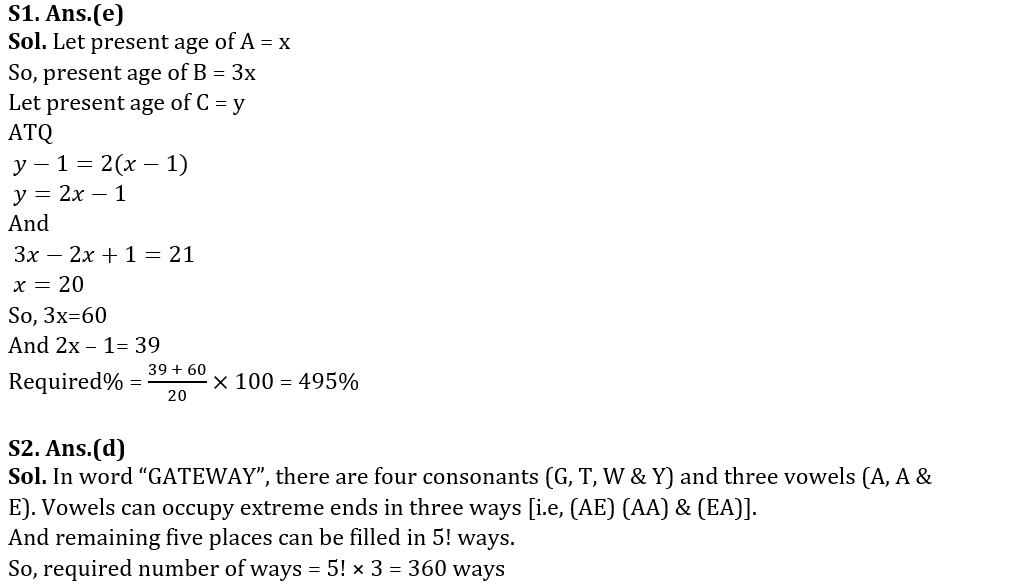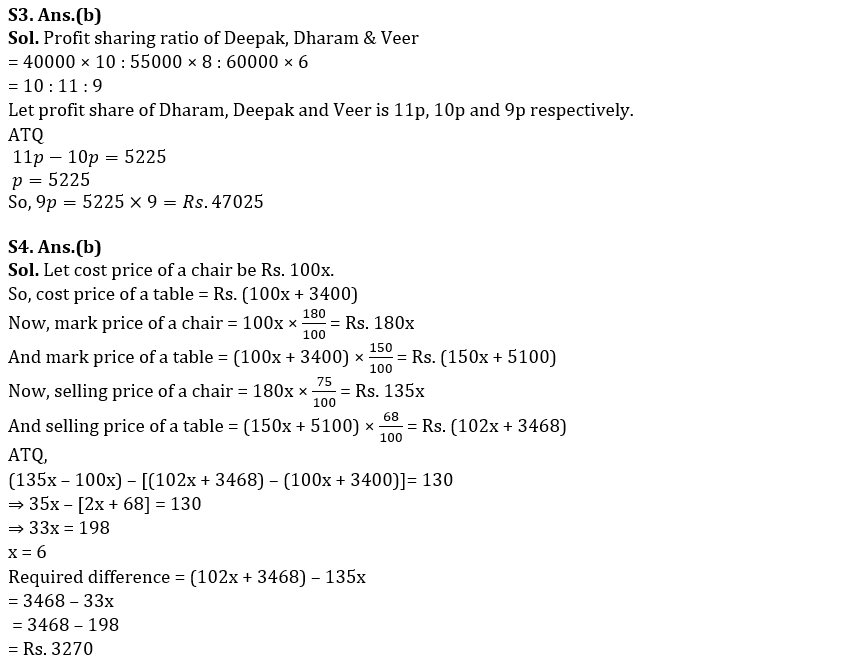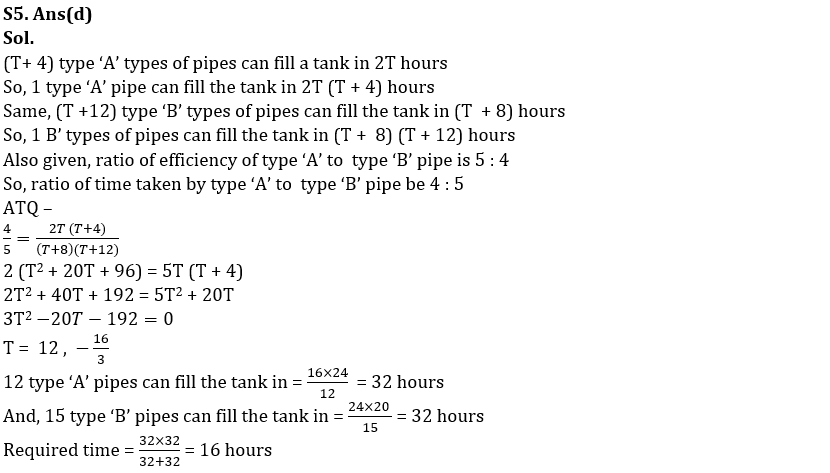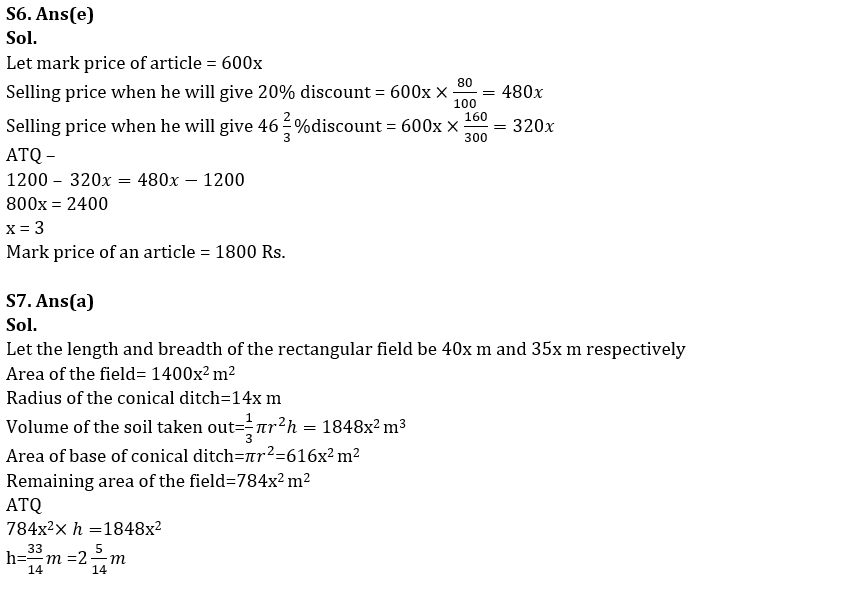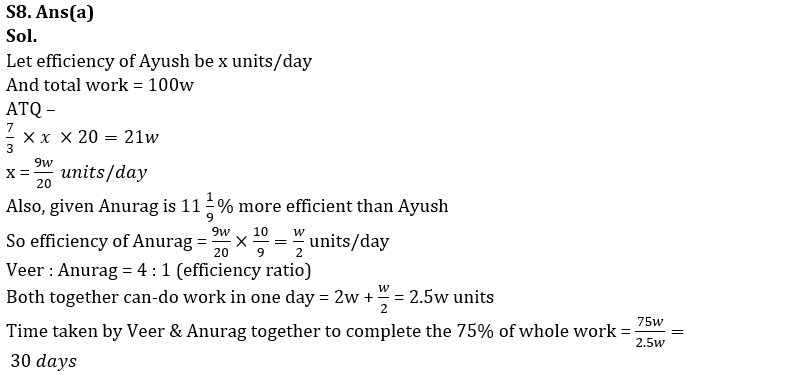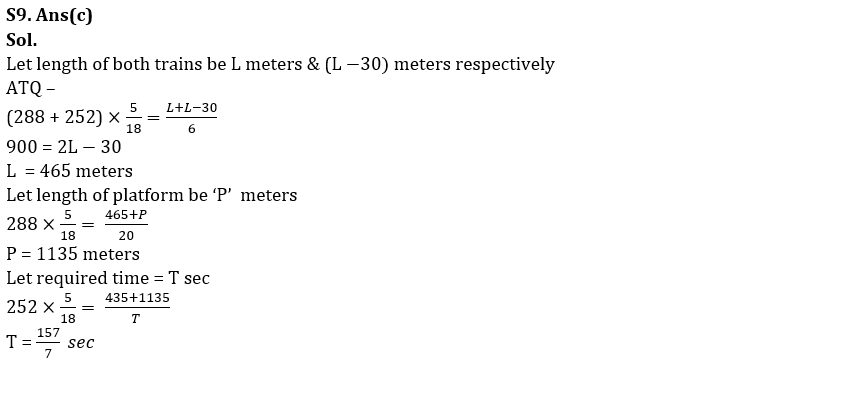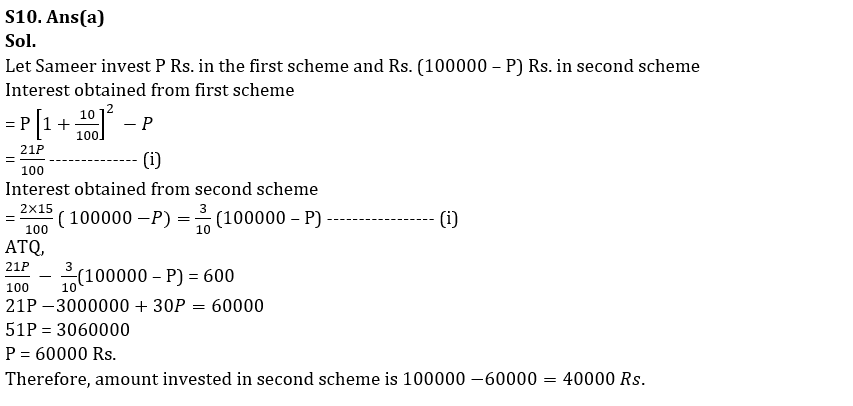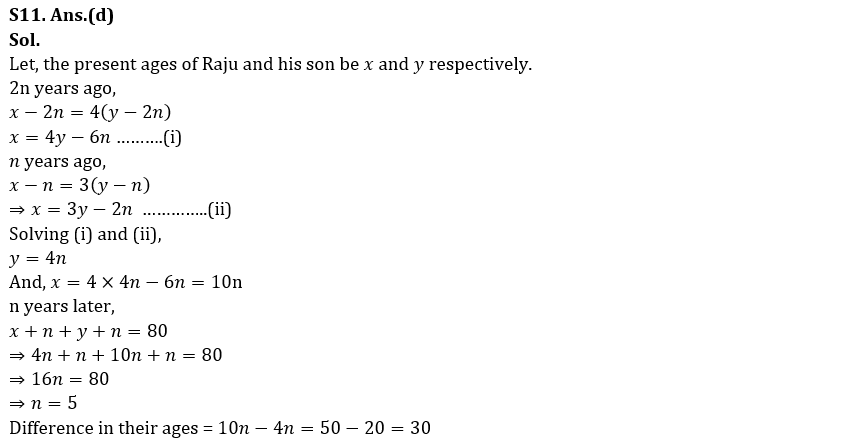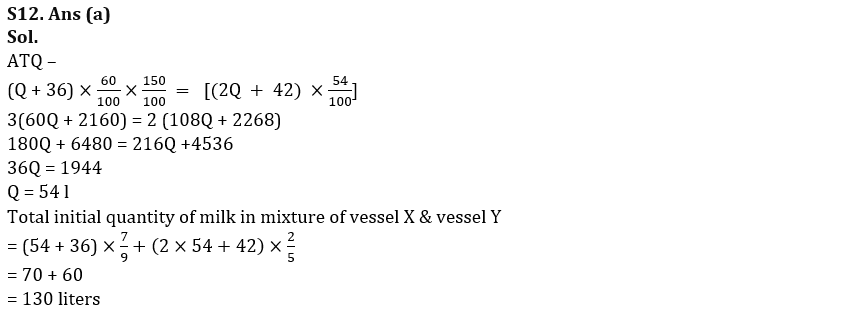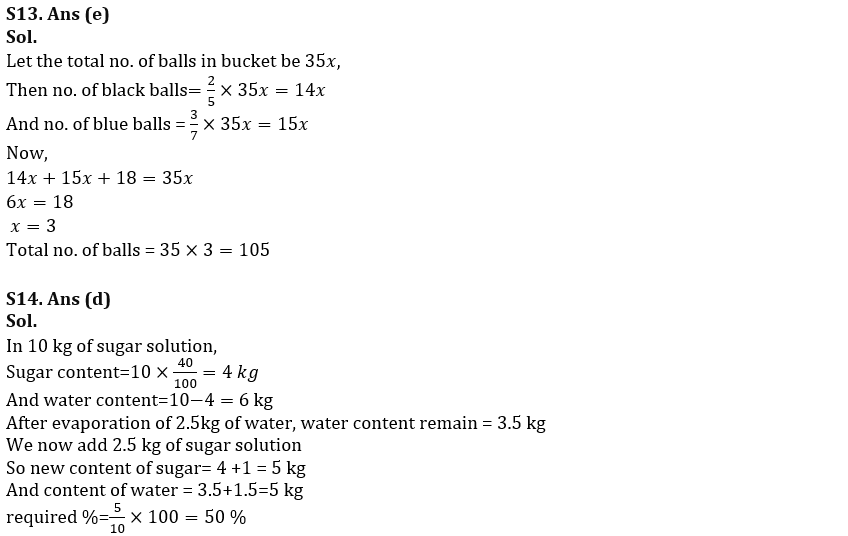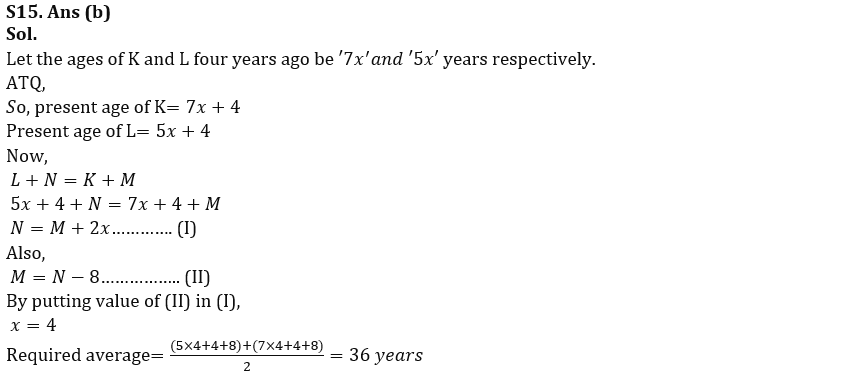## FAQs

### Quantitative Aptitude Quiz For SIDBI Grade A Prelims 2023- 21st January

The SIDBI Grade A Exam 2023 will be held on 28th January 2023.

#### Congratulations!